# Evaluating the Determinant of a Matrix

There are several different ways to solve a system of equations. Another common method is Cranmer’s Rule. However, we cannot learn about Cranmer’s rule until we understand determinants.Determinants are calculated from a square matrix, such as 2×2 or 3×3. In a 2×2 matrix, the determinant is calculating by taking the product of the diagonal and finding the difference.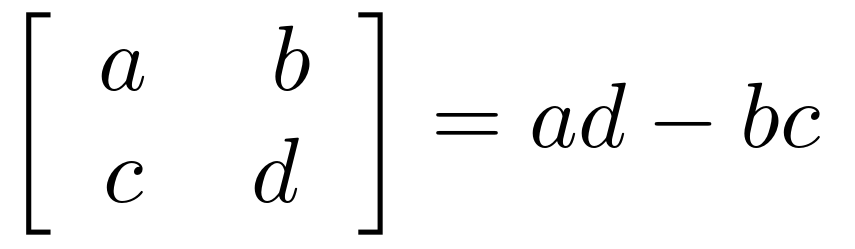Here is how this look with real numbers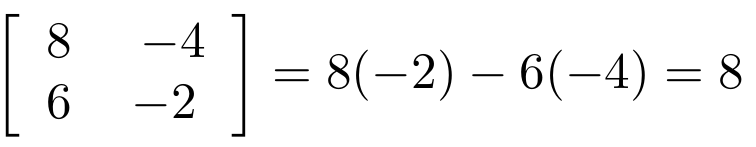Determinant for 3×3 Matrix

To find the determinants of a 3×3 matrix it takes more work.  By address, it is meant the row number and column letter. To calculate the determinant you must remove the row and column of that contains the variable you want to know the determinant too. Doing this creates what is called the minor. Below is an example with variables.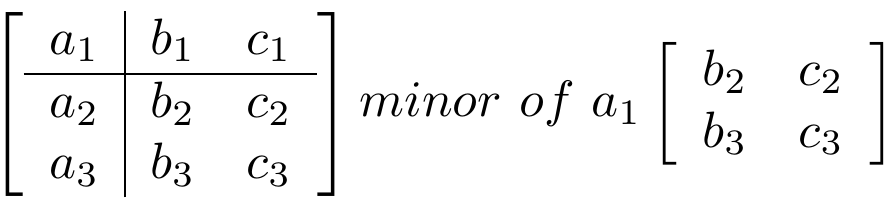As you can see, to find the determinant of a1 we remove the row and the column that contains a1. From there, you do the same math as in a 2×2 matrix. When using real numbers you may need to add the row letter and column number to figure out what you are solving for. Below is an example with real numbers.

Find the determinant of c2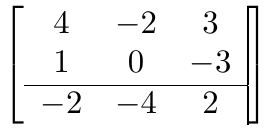The number at c2 is -3. Therefore, we remove the row and the column that contains -3 and we are left with the minor of c2 shown below.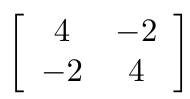IF we follow the steps for a 2×2 matrix we can calculate the determinant of c2 as follows.

## 4(4) – (-2)(-2) = 16 – 12 = 4

Expand by Minors

Knowing the minor is not useful alone, The minor of different columns can be added together to find the determinant for a 3×3 matrix. Below is the expression for finding the determinant of 3×3 matrix.What is happening here is that you find the determinant of a1 and multiply it by the value in a1. You do this again for b1 and c1. Lastly, you find the sum of this process to evaluate the determinant of the 3×3 matrix. Below is another matrix this time with actual numbers. We are going to expand from the first row and first columnAll we do not is obtain the determinant of each 2×2 matrix and multiply it by the outside value before adding it all together. Below is the math.

## 2(-4 – 0) + 3(-6 – 0) – 1(-3 – (2-2)) = -18 – 18 + 1=  -25

Conclusion

This information is not as useful on its own as it is as a precursor to something else. The knowledge acquired here for finding determinants provides us with another way to approach a system of equations using matrices.